Mechanics Index

Simple Machines

Definitions

Any mechanical or electrical device that transmits or modifies energy to perform or assist in the performance of human tasks..
A mechanical device that transmits, modifies, or changes the direction of force in order to help people do work..
A apparatus in which work is done on the machine by applying a force (Effort) at one part which results in work being done by the machine in overcoming an external force (Weight or Resistance). The advantage of using a machine is that a small force can be used to overcome a larger resisting force. Optionally a small movement can be used to cause the machine to generate a larger movement.

There are six basic simple machines..

 Incline Wedge Lever Wheel and Axle Pulley Screw

The Mechanical Advantage of a machine is the ratio of Force being moved W to the Effort F

The Velocity Ratio of a machine is the ratio of the distance moved by the Effort and the distance moved by the Force being overcome.

Velocity Ratio = Distance moved by Effort/ Distance moved by force

In the ideal frictionless/weightless machine

The efficiency of a simple machine

Efficiency = Work done by the machine / Work supplied to machine

The only parameter that can be determined from the machines dimensions is the velocity ratio.

Machines generally follow the linear rule..

F = a + b. W

Incline Plane

One of the simplest of machines is the inclined plane..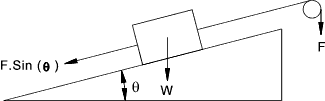The force Force (F) is the effort required to move the Weight (W) up the slope.   A movement of the weight a distance x along the incline will result in a vertical displacement of x Sin(θ)).   Assuming that the incline is frictionless the F required to move the weight up the slope = W Sin(θ)).

Velocity ratio = 1 /Sin(θ)

Wedge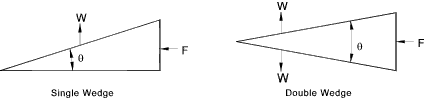Velocity ratio (Single Wedge) = 1 /Tan(θ)
Velocity ratio (Double Wedge) = 2 /Tan(θ/2)

Lever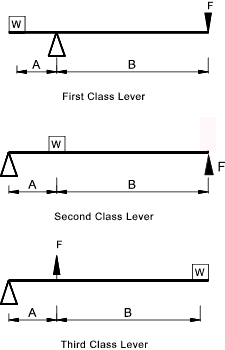Velocity Ratio (First Class Lever) = B /A

Velocity Ratio (Second Class Lever) = (A+B ) /A

Velocity Ratio (Third Class Lever) = A / (A+B )

Wheel and Axle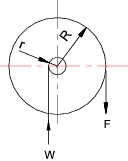Velocity ratio = R / r

Pulley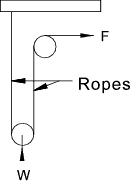Simple Pulley.. Velocity ratio = Number of ropes Note: A very simple 2-rope pulley is shown.  In practice there can be a number of rotating pulleys on the top and bottom blocks increasing the number of vertical ropes.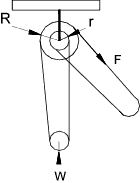Differential Pulley.. Velocity ratio = 2.R /(R-r) Note:Requires use of chain with engaging sprockets to prevent slip

Screw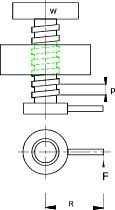Velocity ratio = R. 2.p / p

 Links to Simple Machines Simple Machines in Appliances, Gadgets, and Everyday Life..Lots of relevant papers and links Simple Machines..University Of Arkansas Mysite Machine..A vry informative page of related notes Wikipedia Simple Machines ..Excellent Notes and links

Mechanics Index